# Engineering Mechanics CBGS 2016-2017 BE Computer Engineering Semester 1 (FE First Year) Question Paper Solution

Engineering Mechanics [CBGS]
Date: December 2016

(1)Question no.1 is compulsory.

(2) Attempt any 3 questions from remaining five questions.

1
1.a

Find the force F4 , so as to give the resultant of the force as shown in the figure given below.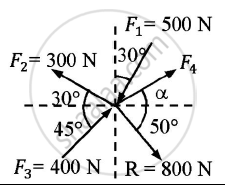Given : Forces and their resultant
To find : Force F4

Concept: Resultant of concurrent forces
Chapter:  System of Coplanar Forces
1.b

A particle starts from rest from origin and it’s acceleration is given by a = k/(x+4)^2     m⁄s^2.Knowing that v = 4 m/s when x = 8m,find :
(1)Value of k
(2)Position when v = 4.5 m/s

Given :  Particle starts from rest
a = k/(x+4)^2    m⁄s^2
v = 4m/s at x = 8m
To find : Value of k and position when v= 4.5m/s

Concept: Introduction to Basic Concepts
Chapter: [11.01] Force and Acceleration
1.c

Rod AB of length 3 m is kept on a smooth plane as shown in the given figure.The velocity of end A is 5 m/s along the inclined plane.Locate the ICR and find velocity of end B.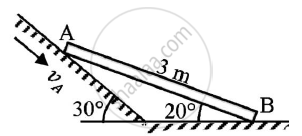Given : Length of rod AB = 3m
va = 5 m/s
To find: ICR
Velocity of end B

Concept: Instantaneous Center of Rotation for the Velocity
Chapter:  Kinematics of Rigid Bodies
1.d

What is a zero force member in a truss? With examples state the conditions for a zero force member.

Concept: Analysis of Plane Trusses by Using Method of Joints
Chapter:  Analysis of Plane Trusses
1.e

A glass ball is dropped on a smooth horizontal floor from which it bounces to a height of 9m.On the second bounce it rises to a height of 6 m.From what height was the ball dropped and find the coefficient of restitution between the glass and the floor.

Given : First bounce height = 9 m
Second bounce height = 6 m
To find : Co-efficient of restitution

Concept: Velocity and Acceleration in Terms of Rectangular Co-ordinate System
Chapter:  Kinematics of Particle
2
2.a

The given figure shows a beam AB hinged at A and roller supported at B. The L shaped portion is welded at D to the beam AB. For the loading shown,find the support reactions.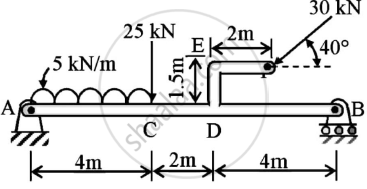Given : Beam AB hinged at A and roller supported at B and different forces acting on it.
To find : Support reactions

Concept: Determination of reactions at supports for various types of loads on beams
Chapter:  Types of Support
2.b

The acceleration time diagram for a linear motion is shown. Construct velocity time diagram and displacement time diagram for the motion assuming that the motion starts with a initial velocity of 5 m/s from the starting point.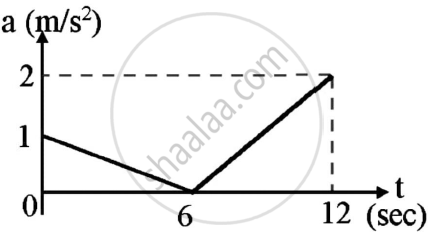Given : Acceleration time graph
To draw : Velocity time graph
Displacement time graph

Concept: Velocity and Acceleration in Terms of Rectangular Co-ordinate System
Chapter:  Kinematics of Particle
2.c

The resultant of the three concurrent space forces at A is 𝑹̅ = (-788𝒋̅) N. Find the magnitude of F1,F2 and F3 forces.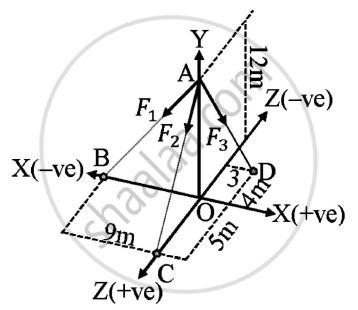Given : A=(0,12,0)
B=(-9,0,0)
C=(0,0,5)
D=(3,0,-4)
Resultant of forces = (-788𝑗̅) N
To find : Magnitude of forces F1,F2,F3

Concept: Resultant of concurrent forces
Chapter:  System of Coplanar Forces
3
3.a

Two spheres A and B of weight 1000N and 750N respectively are kept as shown in the figure..Determine reaction at all contact points 1,2,3 and 4. Radius of A is 400 mm and radius of B is 300 mm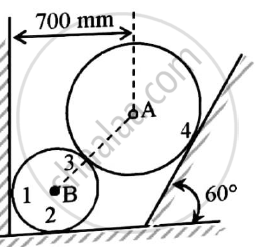Given  : Two spheres are in equilibrium
W1=1000 N
W2=750 N
rA=400 mm
rB=300 mm
To find : Reaction forces at contact points 1,2,3 and 4

Concept: Condition of Equilibrium for non-concurrent nonparallel general forces
Chapter:  Equilibrium of System of Coplanar Forces
3.b

A circle of diameter 1.5 m is cut from a composite plate.Determine the centroid of the remaining area of plate.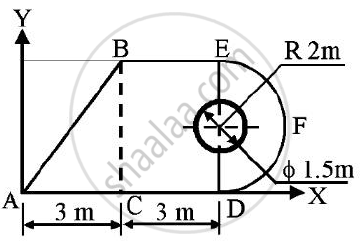Concept: Velocity and Acceleration in Terms of Rectangular Co-ordinate System
Chapter:  Kinematics of Particle
3.c

A rod AB has an angular velocity of 2 rad/sec,counter clock wise as shown.End C of rod BC is free to move on a horizontal surface.Determine:
(1)Angular velocity of rod BC
(2)Velocity of C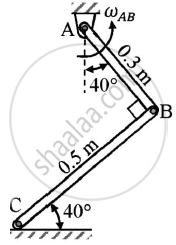Given : ωAB=2 rad/sec (anti clockwise)

To find : ωBC
VC

Concept: Velocity and Acceleration in Terms of Rectangular Co-ordinate System
Chapter:  Kinematics of Particle
4
4.a

A truss is loaded and supported as shown.Determine the following:
(1)Identify the zero force members,if any
(2)Find the forces in members EF,ED and FC by method of joints.
(3)Find the forces in members GF,GC and BC by method of sections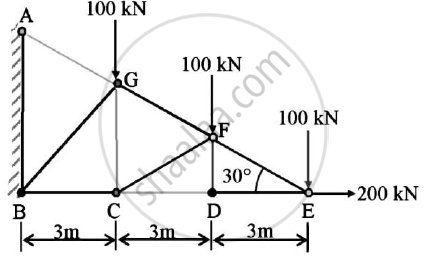Concept: Analysis of Plane Trusses by Using Method of Joints
Chapter:  Analysis of Plane Trusses
4.b

b)A cylinder has a mass of 20kg and is released from rest when h=0 as shown in the figure.Determine its speed when h=3m.The springs have an unstretched length of 2 m.Take k=40 N/m.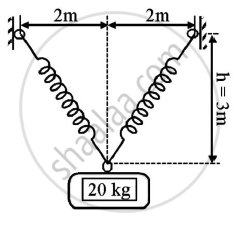Given : m=20 kg
h=0
k=40 N/m
To find : Speed when h=3m

Concept: Principle of Work and Energy
Chapter: [11.02] Work and Energy
4.c

A machine part is subjected to forces as shown.Find the resultant of forces in magnitude and in direction.
Also locate the point where resultant cuts the centre line of bar AB.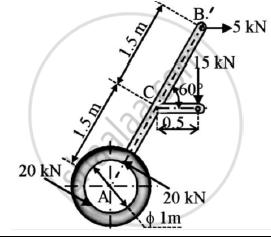Solution:
Given : A machine subjected to various forces
To find : Resultant of forces
Point where the resultant force cuts the bar AB

Concept: Varignon’s Theorem
Chapter:  System of Coplanar Forces
5
5.a

Two blocks A and B are resting against the wall and floor as shown in the figure.Find the minimum value of P that will hold the system in equilibrium. Take μ=0.25 at the floor,μ=0.3 at the wall and μ=0.2 between the blocks.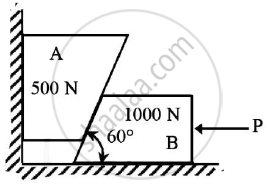Given : μ=0.25 at floor
μ=0.2 between blocks
To find : Minimum value of force P

Concept: Condition of equilibrium for concurrent forces
Chapter:  Equilibrium of System of Coplanar Forces
5.b

A shot is fired with a bullet with an initial velocity of 20 m/s from a point 10 m infront of a vertical wall 5 m high.
Find the angle of projection with the horizontal to enable the shot to just clear the wall. Also find the range of the shot where the bullet falls on the ground.

Given : u=20 m/s
Distance from wall=10m
Height of wall=5m
To find :Angle of projection
Range of shot

Concept: Projectile Motion
Chapter:  Kinematics of Particle
5.c

Three blocks A,B and C of masses 3 kg,2 kg and 7 kg respectively are connected as shown.Determine the acceleration of A,B and C.Also find the tension in the string.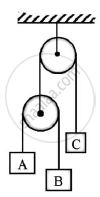Given : mA=3kg
mB=2 kg
mC=7kg
To find: Acceleration of blocks A,B and C

Concept: Condition of equilibrium for parallel forces
Chapter:  Equilibrium of System of Coplanar Forces
6
6.a

Block A of weight 2000N is kept on the inclined plane at 35° .It is connected to weight B by an inextensible string passing over smooth pulley.
Determine the weight of pan B so that B just moves down.Assume μ=0.2.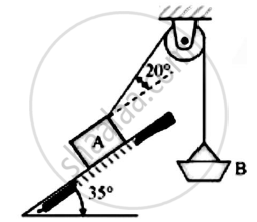Given : Weight of block A=2000N
Angle of inclined plane = 35°
μ=0.2
To find : Weight of pan B

Concept: Condition of equilibrium for parallel forces
Chapter:  Equilibrium of System of Coplanar Forces
6.b

A particle falling under gravity travels 25 m in a particular second. Find the distance travelled by it in the next 3 seconds.

Given : Particle falls 25 m in a particular second
Sn=-25 m
u=0 m/s
To find : Distance travelled by it in next 3 seconds

Concept: Tangentialand Normal Component of Acceleration
Chapter:  Kinematics of Particle
6.c

A rod AD of length 40 cm is suspended from point D as shown in figure. If it has a weight of 25 N and also supports a load of 40N,find the tension in the cable using the method of virtual work.Take AC=30 cm.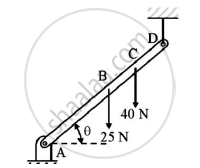Given : Length of rod AD=40cm=0.4m
AC=0.3m
W=25N
To find : Tension in the cable

Concept: Condition of equilibrium for parallel forces
Chapter:  Equilibrium of System of Coplanar Forces

#### Request Question Paper

If you dont find a question paper, kindly write to us

View All Requests

#### Submit Question Paper

Help us maintain new question papers on Shaalaa.com, so we can continue to help students

only jpg, png and pdf files

## University of Mumbai previous year question papers Semester 1 (FE First Year) Engineering Mechanics with solutions 2016 - 2017

University of Mumbai Semester 1 (FE First Year) Engineering Mechanics question paper solution is key to score more marks in final exams. Students who have used our past year paper solution have significantly improved in speed and boosted their confidence to solve any question in the examination. Our University of Mumbai Semester 1 (FE First Year) Engineering Mechanics question paper 2017 serve as a catalyst to prepare for your Engineering Mechanics board examination.
Previous year Question paper for University of Mumbai Semester 1 (FE First Year) Engineering Mechanics-2017 is solved by experts. Solved question papers gives you the chance to check yourself after your mock test.
By referring the question paper Solutions for Engineering Mechanics, you can scale your preparation level and work on your weak areas. It will also help the candidates in developing the time-management skills. Practice makes perfect, and there is no better way to practice than to attempt previous year question paper solutions of University of Mumbai Semester 1 (FE First Year).

How University of Mumbai Semester 1 (FE First Year) Question Paper solutions Help Students ?
• Question paper solutions for Engineering Mechanics will helps students to prepare for exam.
• Question paper with answer will boost students confidence in exam time and also give you an idea About the important questions and topics to be prepared for the board exam.
• For finding solution of question papers no need to refer so multiple sources like textbook or guides.